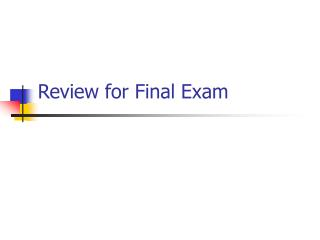DownloadDownload PresentationReview for Final Exam

# Review for Final Exam

Download Presentation## Review for Final Exam

- - - - - - - - - - - - - - - - - - - - - - - - - - - E N D - - - - - - - - - - - - - - - - - - - - - - - - - - -
##### Presentation Transcript

1. Review for Final Exam

2. Schedule • Extra Credit CAPA set now available. • Final exam – Tuesday, April 23 at 7:30 AM in 101 Carroway building. • People who needed to make up a lab – please see me. • People who need to identify their dropped lab, please see me.

3. Final Exam • Electric and Magnetic Forces. • Energy from electric and magnetic forces. • Electric currents. • Simple Circuits • Ohm’s Law. • Kirchhoff’s rules. • Energy, voltages and currents in a circuit • Series and parallel circuits.

4. Final Exam (continued) • Optics • Lens • Snell’s Law • Atoms • Energy Levels • Atomic emission & absorption spectra. • Quantum numbers.

5. Final Exam (continued) • Nuclear Physics • Nuclear reactions • Nuclear energy • Radioactive Decays

6. F = kQ1Q2 R2 Coulomb’s Law R F F +e +e Direction of Electron Forces. • Like charges repel. • Unlike charges attract.

7. Electric Fields The Electric Field is defined as the Force exerted on a tiny positive test charge at that point divided by the magnitude of the test charge: E = F/q E = k q Q/r2 = k Q/r2 [single point charge] q

8. -3.6 C +2.1 C In Class Question How do you find the electric field midway between two point charges? You need to find the vector sum of the two forces. In this case both forces are directed toward the left. Use Coulomb’s Law to find the magnitude of each field separately, then add the two forces to get the magnitude of the net field. E+ = kQ+/R2 E- = kQ-/R2

9. -3.6 C +2.1 C E+ = kQ+/(R2+A2) E- = kQ-/(R2+A2) In Class Question How do you find the electric field midway between two point charges and a distance A above the line the two charges? You need to find the vector sum of the two fields. In this case both fields are not parallel. Use Coulomb’s Law to find the magnitude of each field separately, then add the two forces to get the magnitude of the net field.

10. -3.6 C +2.1 C E+ = kQ+/(R2+A2) E- = kQ-/(R2+A2) In Class Question How do you find the electric field midway between two point charges and a distance A above the line the two charges? In this case the y-components of the fields cancel, and the x-components of the field add. E(total) =kQ-/(R2+A2) cos() + kQ+/(R2+A2) cos()

11. Vab = 1500 V - + +q = .5 C d = 2 mm Electric Potential Energy • PE = PEb - PEa = q(Vb – Va) W = qV = .5 C * 1500 = 750 J W = qV = qEd  E = V/d = 1500 V / 0.002 m = 7.5 x 105 V/m = N/C

12. Sample Problem What is the magnitude of electric force between an iron nuclues (q = +26e) and its innermost electron, if the distance between them is 1.5 x 10-12 m. What direction is the force? What is the electric field due to the nucleus at the location of the electron? What is the electric potential at the location of the electron?

13. Sample Problem An electron (mass m = 9.11×10-31 kg) is accelerated in the uniform field E (E = 1.33×104 N/C) between two parallel charged plates. The separation of the plates is 1.25 cm. The electron is accelerated from rest near the negative plate and passes through a tiny hole in the positive plate, as seen in the figure. With what speed does it leave the hole? F = qE = ma a = qE/m v2 = vo2 + 2a(x-xo) v2 = 2ax = 2(qE/m)x

14. q,v B Forces due to Magnetic Fields • Force on moving charged particles in a magnetic field. • F = qvBsin • Energy is unchanged. The direction is given by the right hand rule. Place fingers of right hand along v. Curl your fingers toward B. Thumb points along F. F is out of the page (q>0).

15. Force on a Current • Force on an electric current in a magnetic field. • Currents consist of moving charges. • F = ILB sin The direction of the force is given by the right-hand rule. Fingers along I. Curl towards B. Thumb points along Force. I  B

16. B-Field Due to Moving Charges. • Moving electric charges produce magnetism. • B = [ o /(4) ] * [ QV/r2 ] • Direction from the right-hand rule. Q, v r B is into the page at this point.

17. B B I I B-Field Due to Currents • Electric currents produce magnetism. • B = oI/(2r) (due to a long straight wire) • Direction from the right-hand rule. Curl your fingers as if following B. Your thumb is in the direction of the current.

18. q,v R B is out of the page. Example: Mass Spectrometer. F = qvB F = ma ma = qvB a = qvB/m qvB/m = v2/R  R = mv/(qB)